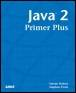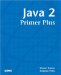# Calling a MethodJava™ 2 Primer PlusBy Steven Haines, Steve Potts Table of Contents Chapter 5.  Methods

After a method is defined, you can call it in your program. In this case the method is publicly available to everyone, so it can be directly referenced inside the main method. The mechanism to call a method is as follows:

` return_datatype variable = method_name( parameter_list ); `

If the method returns a value (the return type is not null), you can assign the result to a variable or use it in any expression that the data type returned by the method is usable. For example, you can use the result of the square method anywhere you can use an int:

` int result = 5 * square( 10 ); // result is 5 * 100 = 500 `

Listing 5.1 shows an example that defines the square method and calls it in a complete Java application.

##### Listing 5.1 Math.java
` 1:  public class Math {  2:    public static int square( int i ) {  3:      return i*i;  4:    }  5:  6:    public static void main( String[] args ) {  7:      for( int i=1; i<=10; i++ ) {  8:         System.out.println( "i=" + i + ", i*i=" + square( i ) );  9:      }  10:   }  11: } `

Line 1 creates a new class named Math.

Lines 2 4 define the square method described in the previous section.

Lines 6 10 define the main method for the Math application. The main method uses a for loop to count from 1 to 10 and prints the current value and the square of the value. The square method invocation is hidden inside the println method call.

The output from the Math application is

` i=1, i*i=1  i=2, i*i=4  i=3, i*i=9  i=4, i*i=16  i=5, i*i=25  i=6, i*i=36  i=7, i*i=49  i=8, i*i=64  i=9, i*i=81  i=10, i*i=100 `

The println method call is pretty busy and could be broken down further and written as follows for clarity:

` int iSquared = square( i );  String output = "i=" + i + ", i*i=" + iSquared;  System.out.println( output ); `

In this example you can see the value returned by the square method call assigned to a variable, and then used. In Listing 5.1 you see that the value returned by the square method is used in a normal expression.

If the method does not return any value then the method call cannot be assigned to a variable or used in an expression. Consider the following method:

` public static void printNumber( int n ) {     System.out.println( "The number is " + n );  } `

Because the method is defined to return void, there is no return type for the method. It could be called as follows:

` int n = 10;  printNumber( n ); // Note the result in not assigned to anything `

 TopJava 2 Primer Plus
ISBN: 0672324156
EAN: 2147483647
Year: 2001
Pages: 332

Similar book on Amazon

flylib.com © 2008-2017.
If you may any questions please contact us: flylib@qtcs.net